Get instant live expert help with Excel or Google Sheets“My Excelchat expert helped me in less than 20 minutes, saving me what would have been 5 hours of work!”

#### Post your problem and you’ll get Expert help in seconds.

Your message must be at least 40 characters
Our professional Expert are available now. Your privacy is guaranteed.

# Excel COLUMN Function

We can use the excel COLUMN function to return the column number for a reference. Column function returns columns as numbers. For instance, Column B is 2 and Column C is 3. Without a reference, COLUMN function will return the column number of the cell containing the formula. The steps below will walk through the process.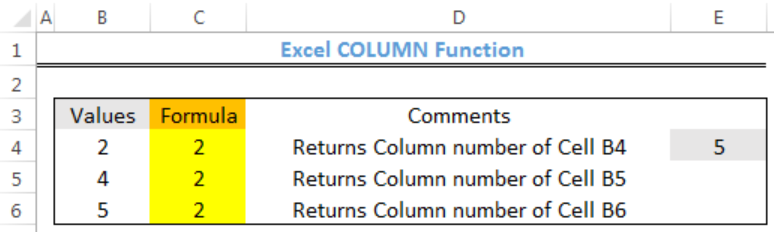Figure 1: How to Use Excel Column Function

## Syntax

`=COLUMN(REFERENCE)`

## Formula

`=COLUMN(B4)`

## Setting up the Data

• We will set up data by inputting our Values in Column B
• The COLUMN Function will return the result in Column C
• We will use Cell E4 without a reference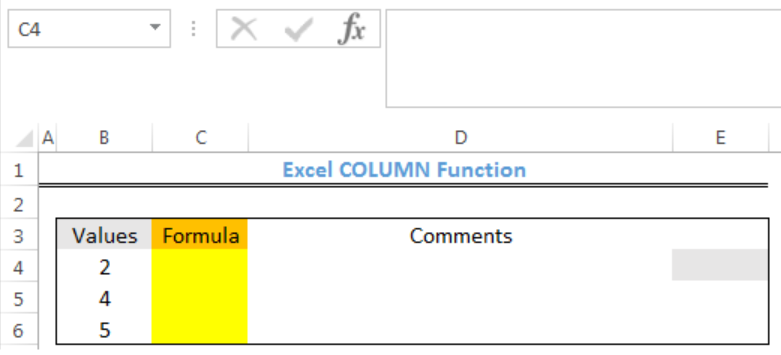Figure 2: Setting up the Data

## Applying the COLUMN Function

• We will click on Cell C4
• We will insert the formula below into the cell
`=COLUMN(B4)`
• We will press the enter key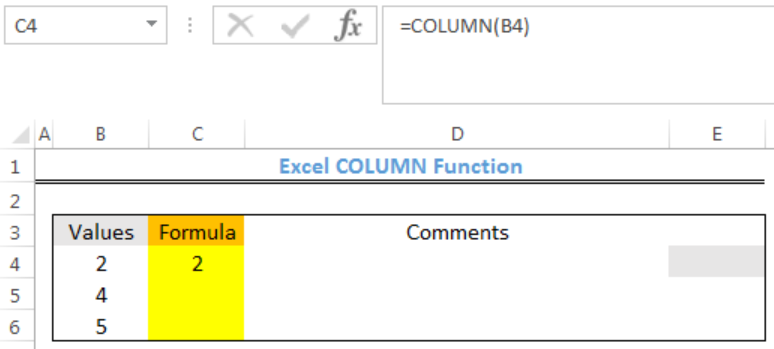Figure 3: Returned Column number for Cell B4

• We will click on Cell C4 again
• We will double click on the fill handle tool which is the small plus sign you see at the bottom right of Cell C4. Select and drag down to copy the formula to other cells.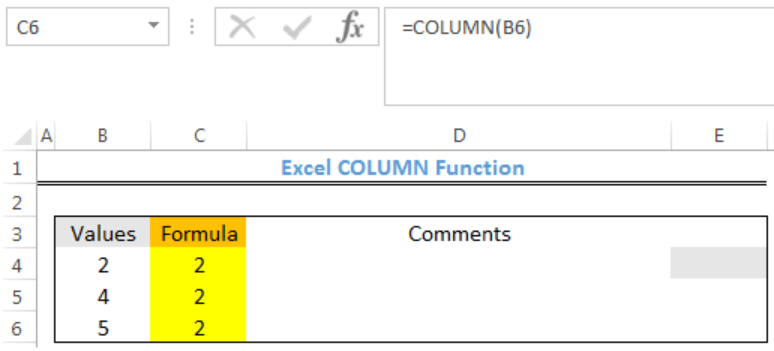Figure 4: Returned Column number for Cell B4 to Cell B6

• We will insert the formula without a reference into Cell E4 and press enter
`=COLUMN()`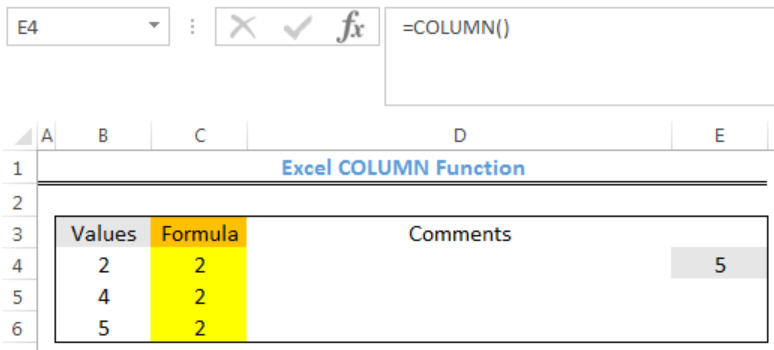Figure 5: Returned Column number without a reference for Cell E4

## Notes

• Column function returns the number of the first column in a range. For instance,=COLUMN(E4:G6) will return 5 as the result because the first column is Column E
• Reference cannot include multiple references or addresses

## Instant Connection to an Expert through our Excelchat Service

Most of the time, the problem you will need to solve will be more complex than a simple application of a formula or function. If you want to save hours of research and frustration, try our live Excelchat service! Our Excel Experts are available 24/7 to answer any Excel question you may have. We guarantee a connection within 30 seconds and a customized solution within 20 minutes.

### Did this post not answer your question? Get a solution from connecting with the expert.Another blog reader asked this question today on Excelchat:
Solution examplesHello, I need help regarding indirect formula of excel. 1. What is the purpose of using this formula? 2. If we use this formula with sum formula, then why we are using this with sum formula, we can also perform addition from different sheets without indirect formula ?
Solved by O. D. in 21 minsneed help how to add a percentage to a column and subtract
Solved by B. U. in 43 minsHello, I need help on sorting a rather large list. It is data with people's names and things they purchased. COLUMN A: Items Purchased COLUMN B: FIRST NAME COLUMN C: LAST NAME For every item purchased, is another row even if it's the same person. So: Doll Abbi Sumandal Toy Abbi Sumandal I'm trying to find a way for Excel to recognize duplicates in columns B&C (Abbi Sumandal) and take the unique values and set them in the same row. Final: Abbi Sumandal Doll Toy Is there a formula or function that can do this?!
Solved by V. H. in 26 minsHOW CAN I MAKE A TALE FOR ONLY 6 COLUMN?
Solved by Z. A. in 40 minsi NEED TO SUMM UP TO ONE THOUSAND ENTRIES IN A COLUMN
Solved by T. W. in 18 mins## Subscribe to Excelchat.coAnother blog reader asked this question today on Excelchat: